In :
# Imports
from __future__ import division
import numpy as np
import matplotlib
import matplotlib.pyplot as plt
import seaborn as sb
import scipy.optimize as opt
from mpl_toolkits.mplot3d import Axes3D, proj3d
from matplotlib.colors import LogNorm

# Magics
%matplotlib inline


# Optimization: An Introduction to the Nelder-Mead Algorithm¶

### Author: Chase Coleman¶

One of the most used optimization algorithms of today is the Nelder-Mead algorithm. It has become a core muscle in many programming languages' minimization techniques, including being the default for both Matlab and Scipy's fmin function. One of its key benefits is that it requires no information about first or second derivatives. The Nelder-Mead algorithm searches for the minimum value of an objective function map $f : \mathbb{R}^{n} \rightarrow \mathbb{R}$ by applying simple operations to a simplex of $n+1$ points in $\mathbb{R}^n$. The algorithm is simple and a basic understanding of it can provide valuable intuition for when it is (and more importantly when it isn't) an appropriate minimization technique.

## Key Components of the Algorithm¶

The algorithm relies on 4 main operations on a simplex of points. Before presenting the main algorithm, we will discuss these operations to simplify the process later. The four operations are:

• Reflection
• Expansion
• Contraction
• Shrink

We will discuss these operations in the context of a concrete example. Consider the following simplex of points in 2-D. Let $\Delta$ be a simplex that consists of the points $x_1 = (0, 0); x_2 = (2, 3); x_3 = (4, 0)$. We can then compute the center of mass $\bar{x} = \frac{1}{3} \sum_{i=1}^3 x_i = (2, 1)$. We graph the points of our simplex and their center of mass below.

In :
alpha, beta, gamma, delta = 1., 2., .5, .75
x1, x2, x3 = np.array([0., 0.]), np.array([2., 3.]), np.array([4., 0.])
xbar = np.array([2, 1])
Delta = np.vstack([x1, x2, x3])
offsets = [(-15, 10), (5, 5), (5, 5)]

# init_simplex = Polygon(Delta, closed=True)
fig, ax = plt.subplots(1, figsize=(10, 8))
ax.set_xlim((-2, 7))
ax.set_ylim((-5, 5))

for ind, point in enumerate(Delta):
curr_x = r"$x_{}$"
curr_offset = offsets[ind]
ax.scatter(point, point, color="k")
ax.annotate(curr_x.format(ind+1), xy=point, xytext=curr_offset,
textcoords='offset points', size=18)

ax.scatter(xbar, xbar, color="k")
ax.annotate(r"$\bar{x}$", xy=xbar, xytext=xbar,
textcoords='offset points', size=16)

plt.show(fig)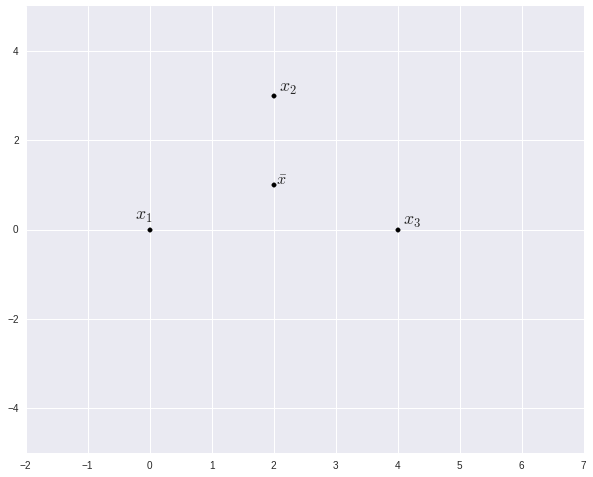To facilitate a simple description of these operations, we sweep several formalities under the rug. First, the point that has these operations applied to it is chosen within the algorithm, but we simply perform all of our operations on $x_2$. Second, and perhaps more importantly, the center of mass used within the algorithm is not the center of mass of all $n+1$ points (it is the center of mass of $n$ points where we exclude the point for which the function ahieves the highest value). Also for aesthetic purposes, we use some nonstandard parameter values in the graphing of these operations ($\alpha = 1$, $\beta = 2$, $\gamma=.5$, and $\delta=.75$).

### Reflection¶

The reflection operation creates a new point defined by reflecting a point across the center of mass of the simplex by $x_r := \bar{x} + \alpha (\bar{x} - x_i)$, where $x_i$ is the point we are reflecting. Thus in our example, $x_r = \bar{x} + \alpha (\bar{x} - x_2) = (2, 1) + \alpha \left( (2, 1) - (2, 3) \right) = (2, -1)$. We add the reflected point below.

In :
xr = xbar + alpha*(xbar - x2)

ax.scatter(xr, xr, color="b")
ax.annotate(r"$x_r$", xy=xr, xytext=xr,
textcoords='offset points', size=16)

fig

Out: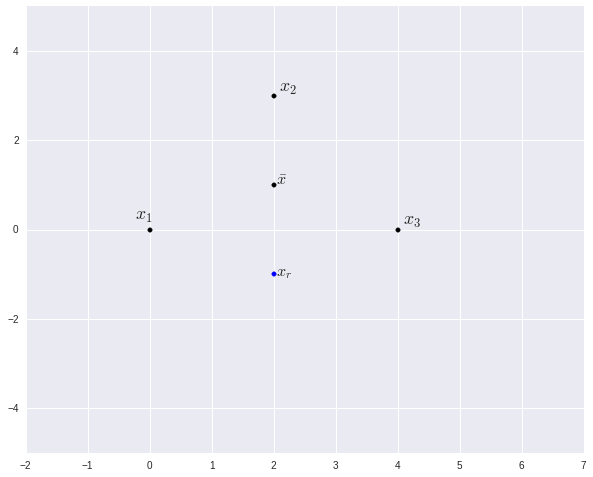### Expansion¶

The expansion operation creates a new point by expanding the reflected point further away from $\bar{x}$. It is created by $x_e := \bar{x} + \beta (x_r - \bar{x})$. We can see in our example that $x_e = \bar{x} + \beta (x_r - \bar{x}) = (2, 1) + \beta \left( (2, -1) - (2, 1) \right) = (2, -3)$. We add the expanded point below.

In :
xe = xbar + beta*(xr - xbar)

ax.scatter(xe, xe, color="g")
ax.annotate(r"$x_e$", xy=xe, xytext=xe,
textcoords='offset points', size=16)

fig

Out: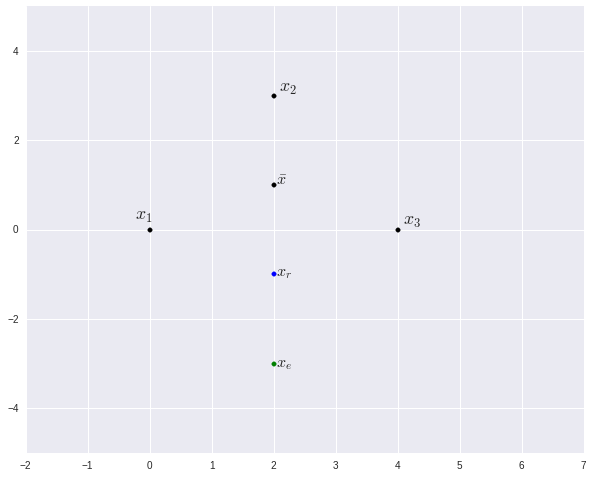### Contraction¶

There are two types of contractions: outside and inside. The operation of contraction is the opposite of the operation expanding in the sense that instead of expanded the reflected point out further, it draws it closer towards the center of mass.

The outside contraction creates a new point by contracting towards the center of mass from the reflected point and is defined by $x_{oc} = \bar{x} + \gamma (x_r - \bar{x})$.

The inside contaction creates a new point by contracting towards the center of mass from the point $x_i$ that we reflected on and is defined by $x_{ic} = \bar{x} + \gamma (x_r - \bar{x})$.

In :
xoc = xbar + gamma*(xr - xbar)
xic = xbar - gamma*(xr - xbar)

ax.scatter(xoc, xoc, color="r")
ax.scatter(xic, xic, color="r")
ax.annotate(r"$x_{oc}$", xy=xoc, xytext=xoc,
textcoords='offset points', size=16)
ax.annotate(r"$x_{ic}$", xy=xic, xytext=xic,
textcoords='offset points', size=16)

fig

Out: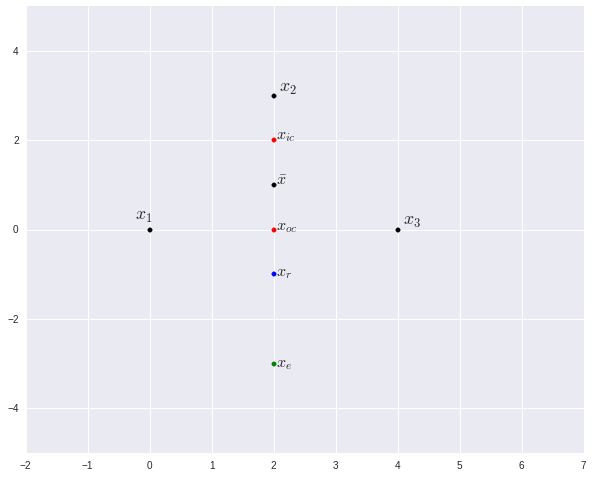### Shrink¶

The shrink operation takes all but one of the points and draws them closer to that one point. In the algorithm, we won't be shrinking the points towards the point that we perform the reflection/expansion/contraction on, so we will use $x_1$ as the point towards which the points "shrink." For every point except $x_1$, we create a new point $x_i^s = x_1 + \delta (x_i - x_1)$.

In :
xs2 = x1 + delta*(x2 - x1)
xs3 = x1 + delta*(x3 - x1)

ax.scatter(xs2, xs2, color="DarkOrange")
ax.scatter(xs3, xs3, color="DarkOrange")
ax.annotate(r"$x_{2}^s$", xy=xs2, xytext=xs2,
textcoords='offset points', size=16)
ax.annotate(r"$x_{3}^s$", xy=xs3, xytext=xs3,
textcoords='offset points', size=16)

fig

Out: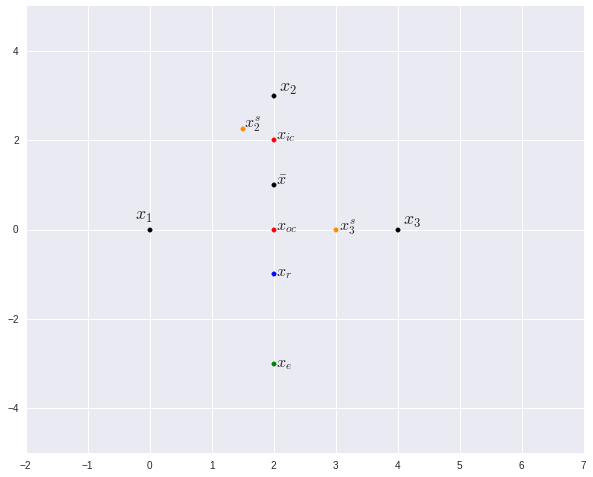Now that we understand what each of the 4 operations within the Nelder-Mead algorithm do, we can discuss the actual algorithm. As you read through the algorithm take note that the main idea is very simple: Order the points, Create some new points, Replace the point with the largest function value, Repeat.

1. There are two ways to obtain the initial simplex. The first way is to simply pass in a simplex $\Delta$ as the guess. The second is to pass in a single point, $x_0$, and create the simplex based around that point (we do this by using $x_0$ as one point of the simplex and by using $x_i := x_0 + e_i \varepsilon$ for the $n$ other points).

2. Once we have the simplex, we evaluate the function at each of the points in the simplex and sort the points such that $x_1 \leq x_2 \leq \dots \leq x_{n+1}$ and find $\bar{x} := \frac{1}{n} \sum_{i=1}^n x_i$ (Notice as we mentioned earlier, we are only taking the center point of the $n$ points with smallest function evaluations).

3. If $|f(\bar{x}) - f(x_1)|$(or another convergence metric of your choosing) then return $x_1$ as the minimum value, otherwise proceed.

4. Create a reflected point $x_r$.

4.1 If $f(x_1) \leq f(x_r) < f(x_n)$ then replace $x_{n+1}$ with $x_r$

5. Else if $f(x_r) < f(x_1)$ then create the expanded point $x_e$.

5.1 If $f(x_e) < f(x_r)$ then replace $x_{n+1}$ with $x_e$

5.2 Else if $f(x_r) < f(x_e)$ then replace $x_{n+1}$ with $x_r$.

6. Else if $f(x_n) < f(x_r) < f(x_{n+1})$ then create the outside contraction point $x_{oc}$.

6.1 If $f(x_{oc}) < f(x_r)$ then replace $x_{n+1}$ with $x_{oc}$

6.2 Else, shrink the points towards $x_1$

7. Else if $f(x_{n+1}) < f(x_r)$ then create the inside contraction point $x_{ic}$.

7.1 If $f(x_{ic}) < f(x_r)$ then replace $x_{n+1}$ with $x_{ic}$

7.2 Else, shrink the points towards $x_1$

That is the entire algorithm. As previously stated, you can see that it simply applies our main operations repeatedly until we converge. I have written a simple implementation of the algorithm below.

In :
"""
Author: Chase Coleman
Date: August 13, 2014

This is a simple implementation of the Nelder-Mead algorithm

"""

def nelder_mead(f, x0, method="ANMS", tol=1e-8, maxit=1e4, iter_returns=None):
"""
This is a naive python implementation of the nelder-mead algorithm.

Parameters
----------

f : callable
Function to minimize
x0 : scalar(float) or array_like(float, ndim=1)
The initial guess for minimizing
method : string or tuple(floats)
If a string, should specify ANMS or NMS then will use specific
parameter values, but also can pass in a tuple of parameters in
order (alpha, beta, gamma, delta), which are the reflection,
expansion, contraction, and contraction parameters
tol : scalar(float)
The tolerance level to achieve convergence
maxit : scalar(int)
The maximimum number of iterations allowed

References :

Nelder, J. A. and R. Mead, "A Simplex Method for Function
Minimization." 1965. Vol 7(4). Computer Journal

F. Gao, L. Han, "Implementing the Nelder-Mead simplex algorithm with

http://www.brnt.eu/phd/node10.html#SECTION00622200000000000000

TODO:
* Check to see whether we can use an array instead of a list of
tuples
* Write some tests
"""
#-----------------------------------------------------------------#
# Set some parameter values
#-----------------------------------------------------------------#
init_guess = x0
fx0 = f(x0)
dist = 10.
curr_it = 0

# Get the number of dimensions we are optimizing
n = np.size(x0)

if method is "ANMS":
alpha = 1.
beta = 1. + (2./n)
gamma = .75 - 1./(2.*n)
delta = 1. - (1./n)
# Otherwise can use standard parameters
elif method is "NMS":
alpha = 1.
beta = 2.
gamma = .5
delta = .5
elif type(method) is tuple:
alpha, beta, gamma, delta = method

#-----------------------------------------------------------------#
# Create the simplex points and do the initial sort
#-----------------------------------------------------------------#
simplex_points = np.empty((n+1, n))

pt_fval = [(x0, fx0)]

simplex_points[0, :] = x0

for ind, elem in enumerate(x0):

if np.abs(elem) < 1e-14:
curr_tau = 0.00025
else:
curr_tau = 0.05

curr_point = np.squeeze(np.eye(1, M=n, k=ind)*curr_tau + x0)

simplex_points[ind, :] = curr_point
pt_fval.append((curr_point, f(curr_point)))

if iter_returns is not None:
ret_points = []
else:
ret_points = None

#-----------------------------------------------------------------#
# The Core of The Nelder-Mead Algorithm
#-----------------------------------------------------------------#
while dist>tol and curr_it<maxit:

# 1: Sort and find new center point (excluding worst point)
pt_fval = sorted(pt_fval, key=lambda v: v)
xbar = x0*0

for i in range(n):
xbar = xbar + (pt_fval[i])/(n)

if iter_returns is not None and curr_it in iter_returns:
ret_points.append(pt_fval)

# Define useful variables
x1, f1 = pt_fval
xn, fn = pt_fval[n-1]
xnp1, fnp1 = pt_fval[n]

# 2: Reflect
xr = xbar + alpha*(xbar - pt_fval[-1])
fr = f(xr)

if f1 <= fr < fn:
# Replace the n+1 point
xnp1, fnp1 = (xr, fr)
pt_fval[n] = (xnp1, fnp1)

elif fr < f1:
# 3: expand
xe = xbar + beta*(xr - xbar)
fe = f(xe)

if fe < fr:
xnp1, fnp1 = (xe, fe)
pt_fval[n] = (xnp1, fnp1)
else:
xnp1, fnp1 = (xr, fr)
pt_fval[n] = (xnp1, fnp1)

elif fn <= fr <= fnp1:
# 4: outside contraction
xoc = xbar + gamma*(xr - xbar)
foc = f(xoc)

if foc <= fr:
xnp1, fnp1 = (xoc, foc)
pt_fval[n] = (xnp1, fnp1)
else:
# 6: Shrink
for i in range(1, n+1):
curr_pt, curr_f = pt_fval[i]
# Shrink the points
new_pt = x1 + delta*(curr_pt - x1)
new_f = f(new_pt)
# Replace
pt_fval[i] = new_pt, new_f

elif fr >= fnp1:
# 5: inside contraction
xic = xbar - gamma*(xr - xbar)
fic = f(xic)

if fic <= fr:
xnp1, fnp1 = (xic, fic)
pt_fval[n] = (xnp1, fnp1)
else:
# 6: Shrink
for i in range(1, n+1):
curr_pt, curr_f = pt_fval[i]
# Shrink the points
new_pt = x1 + delta*(curr_pt - x1)
new_f = f(new_pt)
# Replace
pt_fval[i] = new_pt, new_f

# Compute the distance and increase iteration counter
dist = abs(fn - f1)
curr_it = curr_it + 1

if curr_it == maxit:
raise ValueError("Max iterations; Convergence failed.")

if ret_points:
return x1, f1, curr_it, ret_points
else:
return x1, f1, curr_it


## Example: Rosenbrock Function¶

One of the key tests for an optimization algorithm is Rosenbrock's "banana function" which is $f(x, y) := (a - x)^2 + b(y - x^2)^2$ which has a minimum at $(a, a^2)$. It is a tricky function because of nonconvexities and there are many points that are close to being a minimium. I graph the function below:

In :
# Define Rosenbrock Function
def rosenbrock(x, a=1, b=100):
"""
The minimum value of rosenbrock function is
(a, a**2)
"""
y = x
x = x
return (a - x)**2 + b*(y - x**2)**2

In [ ]:


In :
x = np.linspace(-2.5, 2.5, 500)
y = np.linspace(-2.5, 2.5, 500)

X, Y = np.meshgrid(x, y)

Z = rosenbrock([X, Y])

fig = plt.figure(figsize=(14, 8))

fig.suptitle("Rosenbrock Function", size=24)

# Color mesh
ax1.set_axis_bgcolor("white")
ax1.pcolormesh(X, Y, Z, cmap=matplotlib.cm.viridis,
norm=LogNorm())
ax1.scatter(1, 1, color="k")
ax1.annotate('Global Min', xy=(1, 1), xytext=(-0.5, 1.25),
arrowprops=dict(facecolor='black', shrink=0.05))

# Surface plot
ax2.set_axis_bgcolor("white")
ax2.plot_surface(X, Y, Z, norm = LogNorm(), cmap=matplotlib.cm.viridis,
linewidth=0)
ax2.view_init(azim=65, elev=25)
ax2.scatter(1., 1., 0., color="k")
xa, ya, _ = proj3d.proj_transform(1,1,0, ax2.get_proj())
ax2.annotate("Global Min", xy = (xa, ya), xytext = (-20, 30),
textcoords = 'offset points', ha = 'right', va = 'bottom',
arrowprops=dict(facecolor='black', shrink=0.05))

plt.tight_layout()
plt.show()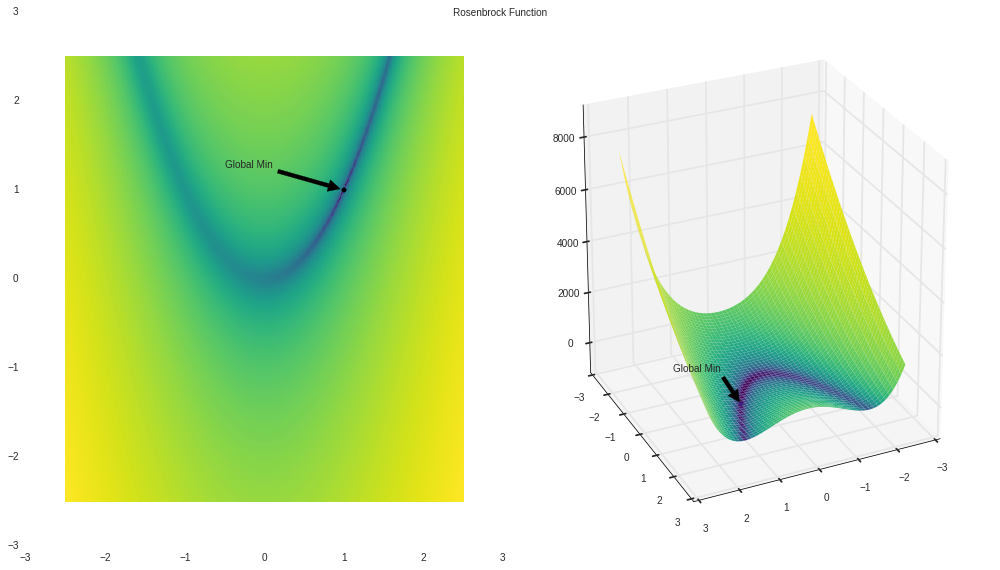# Performance of the Nelder Mead¶

Now that we have seen the objective function, I will try and use our algorithm to find the minimum of this function. To show the progress, I will plot some of the steps below --In particular, I will plot iterations 0, 1, 2, 3, 4, 5, 10, 20, 50, 75, 90, and 95 as set by iterstosee.

In :
iterstosee = [0, 1, 2, 3, 4, 5, 10, 15, 30, 45, 75, 90]
x, fx, its, ret_tris = nelder_mead(rosenbrock, x0=np.array([-1.5, -1.]), tol=1e-12, iter_returns=iterstosee)

fig, axs = plt.subplots(nrows=6, ncols=2, figsize=(16, 24))
axs = axs.flatten()

# Color mesh
for i, curr_ax in enumerate(axs):
curr_simplex = np.vstack([ret_tris[i], ret_tris[i], ret_tris[i]])
curr_ax.pcolormesh(X, Y, Z, cmap=matplotlib.cm.viridis,
norm=LogNorm())
curr_ax.set_title("This is simplex for iteration %i" %iterstosee[i])
curr_ax.scatter(curr_simplex[:, 0], curr_simplex[:, 1])

plt.tight_layout()
plt.show()